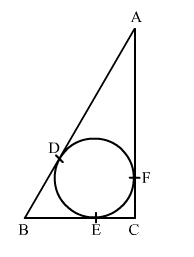# In the given figure, a circle inscribed in a triangle ABC, touches the sides AB, BC and AC at point D,`
Question:

In the given figure, a circle inscribed in a triangle ABC, touches the sides AB, BC and AC at point D, E and F respectively. If AB = 14 cm, BC = 8 cm and AC = 12 cm. Find the lengths of AD, BE and CFSolution:

We know that tangent segments to a circle from the same external point are congruent.
Now, we have
AD = AF, BD = BE and CE = CF
Now, AD + BD = 14 cm          .....(1)
AF + FC = 12 cm
⇒ AD + FC = 12 cm                    .....(2)
BE + EC = 8 cm
⇒ BD + FC = 8 cm                   .....(3)